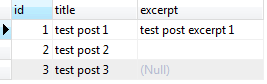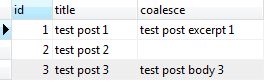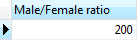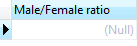# PostgreSQL Tutorial: NULLIF

August 4, 2023

Summary: this tutorial shows you how to use PostgreSQL NULLIF function to handle null values. We will show you some examples of using the NULLIF function.

## PostgreSQL NULLIF function syntax

The `NULLIF` function is one of the most common conditional expressions provided by PostgreSQL. The following illustrates the syntax of the `NULLIF` function:

``````NULLIF(argument_1,argument_2);
``````

The `NULLIF` function returns a null value if `argument_1` equals to `argument_2`, otherwise it returns `argument_1`.

See the following examples:

``````SELECT
NULLIF (1, 1); -- return NULL

SELECT
NULLIF (1, 0); -- return 1

SELECT
NULLIF ('A', 'B'); -- return A
``````

## PostgreSQL NULLIF function example

Let’s take a look at an example of using the `NULLIF` function.

First, we create a table named `posts` as follows:

``````CREATE TABLE posts (
id serial primary key,
title VARCHAR (255) NOT NULL,
excerpt VARCHAR (150),
body TEXT,
created_at TIMESTAMP DEFAULT CURRENT_TIMESTAMP,
updated_at TIMESTAMP
);
``````

Second, we insert some sample data into the posts table.

``````INSERT INTO posts (title, excerpt, body)
VALUES
('test post 1','test post excerpt 1','test post body 1'),
('test post 2','','test post body 2'),
('test post 3', null ,'test post body 3');
``````

Third, our goal is to display the posts overview page that shows title and excerpt of each posts. In case the excerpt is not provided, we use the first 40 characters of the post body. We can simply use the following query to get all rows in the `posts` table.

``````SELECT
ID,
title,
excerpt
FROM
posts;
``````We see the null value in the excerpt column. To substitute this null value, we can use the COALESCE function as follows:

``````SELECT
id,
title,
COALESCE (excerpt, LEFT(body, 40))
FROM
posts;
``````Unfortunately, there is mix between null value and ” (empty) in the excerpt column. This is why we need to use the `NULLIF` function:

``````SELECT
id,
title,
COALESCE (
NULLIF (excerpt, ''),
LEFT (body, 40)
)
FROM
posts;
``````

Let’s examine the expression in more detail:

• First, the `NULLIF` function returns a null value if the excerpt is empty, otherwise it returns the excerpt. The result of the `NULLIF` function is used by the `COALESCE` function.
• Second, the `COALESCE` function checks if the first argument, which is provided by the `NULLIF` function, if it is null, then it returns the first 40 characters of the body; otherwise it returns the excerpt in case the excerpt is not null.

## Use NULLIF to prevent division-by-zero error

Another great example of using the `NULLIF` function is to prevent division-by-zero error. Let’s take a look at the following example.

First, we create a new table named members:

``````CREATE TABLE members (
ID serial PRIMARY KEY,
first_name VARCHAR (50) NOT NULL,
last_name VARCHAR (50) NOT NULL,
gender SMALLINT NOT NULL -- 1: male, 2 female
);
``````

Second, we insert some rows for testing:

``````INSERT INTO members (
first_name,
last_name,
gender
)
VALUES
('John', 'Doe', 1),
('David', 'Dave', 1),
('Bush', 'Lily', 2);
``````

Third, if we want to calculate the ratio between male and female members, we use the following query:

``````SELECT
(SUM (
CASE
WHEN gender = 1 THEN
1
ELSE
0
END
) / SUM (
CASE
WHEN gender = 2 THEN
1
ELSE
0
END
) ) * 100 AS "Male/Female ratio"
FROM
members;
``````

To calculate the total number of male members, we use the SUM function and CASE expression. If the gender is 1, the `CASE` expression returns 1, otherwise it returns 0; the `SUM` function is used to calculate total of male members. The same logic is also applied for calculating the total number of female members.

Then the total of male members is divided by the total of female members to return the ratio. In this case, it returns 200%, which is correct.Fourth, let’s remove the female member:

``````DELETE
FROM
members
WHERE
gender = 2;
``````

And execute the query to calculate the male/female ratio again, we got the following error message:

``````ERROR:  division by zero
``````

The reason is that the number of female is zero. To prevent this division by zero error, we use the `NULLIF` function as follows:

``````SELECT
(
SUM (
CASE
WHEN gender = 1 THEN
1
ELSE
0
END
) / NULLIF (
SUM (
CASE
WHEN gender = 2 THEN
1
ELSE
0
END
),
0
)
) * 100 AS "Male/Female ratio"
FROM
members;
``````

The `NULLIF` function checks if the number of female members is zero, it returns null. The total of male members is divided by a null value returns a null value, which is correct.In this tutorial, we have shown you how to apply the NULLIF function to substitute the null values for displaying data and preventing division by zero error.